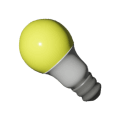Grade 11 Math Light bulb usage | BCHydro Power Smart for Schools
Electricity

# How does your use of lights measure up?

Determine if your household usage of light bulbs is above or below average.11Duration
20 minsType
Thought starter

## Overview

After making an initial conjecture about how the number of light bulbs in their home compares to the average number of lights, students analyze class data using measures of variance (central tendency and standard deviation). Students then use this mathematical evidence to revisit their initial conjecture and draw a sound mathematical conclusion about the number of light bulbs in their home.

### Instructions

#### What you'll need

• "Above or below average?" worksheet
• "Light bulb inventory" worksheet

1. Provide each of your students with a copy of the "Above or below average?" worksheet. Invite them to make an initial conjecture about how the number of lights in their home compares to the average number of lights in their classroom.
2. Provide each student with a copy of the "Light bulb inventory" worksheet. Invite students to do a mental walk-through of their homes, recording the number of light bulbs in their home.
3. Encourage students to share their number with the class. Prompt students to note the numbers offered by their classmates on the "Above or below average?" worksheet.
4. Ask students to complete the table and calculations on the worksheet to gather mathematical evidence of distribution and variance.
5. Invite students to revisit their initial conjecture, this time thinking about the mathematical evidence they collected and calculated.
6. Encourage students to compare their initial conjecture with this new conclusion and to share their observations with the class.

### Modify or extend this activity

#### Modification

• Students could use a spreadsheet or technology to perform the calculations.

#### Extensions

• Students could consider the lighting usage of households in other communities, parts of the province or country, or world to explore the difference between data sampling and populations.
• Students could record the amount of time each light bulb in their household is on during a typical day and determine the average amount of energy used by each light bulb and the standard deviation.

### Curriculum Fit

#### Content

• Applications of Statistics:
• Variation occurs in observation
• How do we analyze data and make decisions?
• How would you describe variation?
• When analyzing data, what are some factors that need to be considered before making an inference?
• Posing a question about an observed variation, collecting and interpreting data, to answer the question
• Measures of central tendency, standard deviation, z-scores and distribution

#### Curricular competencies

##### Reasoning and modelling
• Explore, analyze, and apply mathematical ideas using reason, technology, and other tools
##### Understanding and solving
• Visualize to create and use mental images to support understanding
• Develop, demonstrate and apply mathematical understanding through inquiry and problem-solving
##### Connecting and reflecting
• Reflect on mathematical thinking
• Connect mathematical concepts with each other, other areas, and personal interests
• Use mistakes as opportunities to advance learning

### Teaching Notes

To complete this activity, students would need to understand the concepts of central tendency and standard deviation.

### Assessment

Throughout the activity consider how well students:

• Understand the statistical concept of variance and measures of mean and standard deviation
• Connect personal experiences within a broader context (the community)
• Use appropriate vocabulary (e.g., variance, mean, and standard deviation) in their explanations
• Pay close attention to appropriate details
• Use mathematical evidence to make sound mathematical decisions or conclusions